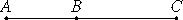# Proposition 36

If two rational straight lines commensurable in square only are added together, then the whole is irrational; let it be called binomial.

Let two rational straight lines AB and BC commensurable in square only be added together.

I say that the whole AC is irrational.X.11

Since AB is incommensurable in length with BC, for they are commensurable in square only, and AB is to BC as the rectangle AB by BC is to the square on BC, therefore the rectangle AB by BC is incommensurable with the square on BC.

But twice the rectangle AB by BC is commensurable with the rectangle AB by BC, and the sum of the squares on AB and BC is commensurable with the square on BC, for AB and BC are rational straight lines commensurable in square only, therefore twice the rectangle AB by BC is incommensurable with the sum of the squares on AB and BC.

And, taken jointly, twice the rectangle AB by BC together with the squares on AB and BC, that is, the square on AC, is incommensurable with the sum of the squares on AB and BC.

X.Def.I.4

But the sum of the squares on AB and BC is rational, therefore the square on AC is irrational, so that AC is also irrational. Let it be called binomial.

Q.E.D.

## Guide

Translated into statements about rational numbers, this proposition says that if a2 and b2 are rational numbers, but a/b is an irrational number, then (a + b)2 is an irrational number. That’s so since (a + b)2 = a2 + 2ab + b2, and ab = (a/bb2.

We can also interpret it as follows where c is a2, and d is b2:

If c and d are rational numbers, but √(c/d) is an irrational number, then (√c + √d)2 is also an irrational number, and √c + √d is called a binomial.

#### Use of this proposition.

This proposition is used very frequently in Book X starting with the next proposition.